# Faster persistent data structures through hashing

November 30, 2011

• Started using Haskell in 2005.

• Worked on (among other things) GHC's new scalable I/O implementation.

# This lecture

• We will design a new data structure and learn how to write high performance Haskell code, at the same time.

• We will revisit some optimization techniques covered earlier, but through a different lens (and with pretty pictures.)

# Motivating problem: Twitter data analysis

"I'm computing a communication graph from Twitter data and then scan it daily to allocate social capital to nodes behaving in a good karmic manner. The graph is culled from 100 million tweets and has about 3 million nodes."

We need a data structure that is

• fast when used with string keys, and

• doesn't use too much memory.

• `Data.Map` is the most commonly used map type.

• It's implemented using size balanced trees and its performance is representative of binary tree implementations (e.g. AVL trees, red-black trees).

• Keys can be of any type, as long as values of the type can be ordered.

# Real world performance of Map

• Good in theory: no more than O(logn) comparisons.

• Not great in practice: up to O(logn) comparisons!

• Many common types are expensive to compare e.g `String`, `ByteString`, and `Text`.

• Given a string of length k, we need O(k * logn) comparisons to look up an entry.

# Hash tables

• Hash tables perform well with string keys: O(k) amortized lookup time for strings of length k.

• Programs using persistent maps are easier to reason about than programs using mutable hash tables, so we'd like to use the former if at all possible.

# Main idea: IntMap as a sparse array

• We can use hashing without using hash tables!

• `Data.IntMap` is much faster than `Map`, but only works with `Int` keys. It's implemented using radix trees (aka Patricia tries).

• An `IntMap` can be used as a persistent, sparse array.

• We can use hashing to derive an `Int` from an arbitrary key:

``class Hashable a where  hash :: a -> Int``
• Lookup using hashing is O(k + logn) instead of O(k * logn).

# Aside: collisions are easy to deal with

• `IntMap` implements a sparse, persistent array of size 232 (or 264).

• Hashing using this many buckets makes collisions rare: for 224 entries we expect about 32,000 single collisions.

• Implication: We can use any old collision handling strategy (e.g. chaining using linked lists).

# `HashMap` implemented using an `IntMap`

Naive implementation:

``newtype HashMap k v = HashMap (IntMap [(k, v)])``

We use a list of key-value pairs to handle collisions.

Knowing how GHC represents values in memory is useful because

• it allows us to approximate memory usage, and

• it allows us to count the number of indirections, which affect cache behavior.

# Memory usage for data constructors

Rule of thumb: a constructor uses one word for a header, and one word for each field. So e.g.

``data Uno = Uno adata Due = Due a b``

an `Uno` takes 2 words, and a `Due` takes 3.

• Exception: a constructor with no fields (like `Nothing` or `True`) takes no space, as it's shared among all uses.

# Memory layout

Here's how GHC represents the list `[1,2]` in memory: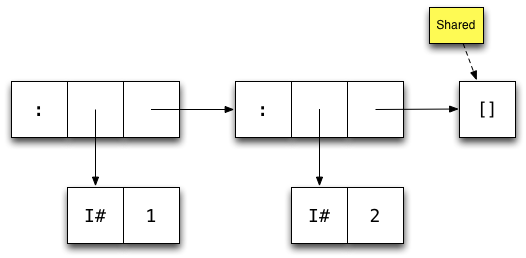• Each box represents one machine word

• Arrows represent pointers

• Each constructor has one word overhead for e.g. GC information

# Refresher: unboxed types

GHC defines a number of unboxed types. These typically represent primitive machine types.

• By convention, the names of these types end with a `#`.

• Most unboxed types take one word (except e.g. `Double#` on 32-bit machines)

• Values of unboxed types cannot be thunks.

• The basic types are defined in terms unboxed types e.g.

``data Int = I# Int#``
• We call types such as `Int` boxed types

# Poll

How many machine words is needed to store a value of this data type:

``data IntPair = IP Int Int``
• 3?

• 5?

• 7?

• 9?

Tip: Draw a boxes-and-arrows diagram.

# IntPair memory layout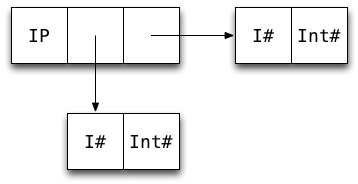So an `IntPair` value takes 7 words.

# Refresher: unpacking

GHC gives us some control over data representation via the `UNPACK` pragma.

• The pragma unpacks the contents of a constructor into the field of another constructor, removing one level of indirection and one constructor header.

• Only fields that are strict, monomorphic, and single-constructor can be unpacked.

The pragma is added just before the bang pattern:

``data Foo = Foo {-# UNPACK #-} !SomeType``

GHC 7 and later will warn if an `UNPACK` pragma cannot be used because it fails the use constraint.

# Unpacking example

``data IntPair = IP !Int !Int````data IntPair = IP {-# UNPACK #-} !Int                  {-# UNPACK #-} !Int``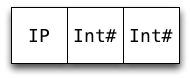# Benefits of unpacking

When the pragma applies, it offers the following benefits:

• Reduced memory usage (4 words saved in the case of `IntPair`)

• Removes indirection

Caveat: There are (rare) cases where unpacking hurts performance e.g. if the value is passed to a non-strict function, as it needs to be reboxed.

Unpacking is one of the most important optimizations available to us.

# A structural comparison with C

By reference:

``-- Haskelldata A = A !Int``
``// Cstruct A {  int *a;};``

By value:

``-- Haskelldata A = A {-# UNPACK #-} !Int``
``// Cstruct A {  int a;};``

If you can figure out which C representation you want, you can figure out which Haskell representation you want.

# Exercise: HashMap memory layout

Here are the data types used in our naive `HashMap` implementation:

``newtype HashMap k v = HashMap (IntMap [(k, v)])data IntMap a    = Bin {-# UNPACK #-} !SuffixMask          !(IntMap a)          !(IntMap a)    | Tip {-# UNPACK #-} !Key a    | Niltype SuffixMask = Inttype Key = Int``

Exercise:

• Draw a diagram of a map containing two key-value pairs of type `Int` (i.e. `Bin ... (Tip ...) (Tip ...)`).

• How many words of memory does the map use?

# Solution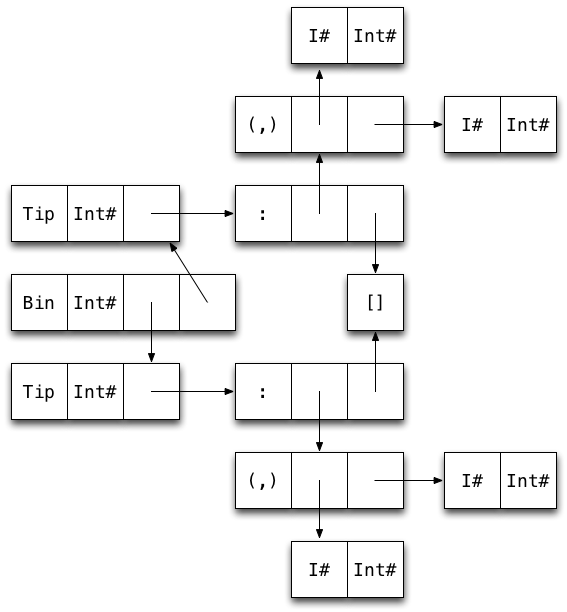30 words! 22 (73%) of them overhead.

# Can we do better?

Yes! We can make use of the following:

• The list of collisions is never empty (and almost always contains a single element).

• We don't need to store arbitrary elements in the list of collisions, just pairs:

``data List k v = Nil | Cons k v (List k v)``

is more memory efficient than `[(k, v)]`, as the pair constructor has been unpacked into the `Cons` constructor.

# An improved HashMap data type

``data HashMap k v    = Bin {-# UNPACK #-} !SuffixMask          !(HashMap k v)          !(HashMap k v)    | Tip {-# UNPACK #-} !Hash          {-# UNPACK #-} !(FullList k v)  -- now monomorphic    | Niltype SuffixMask = Inttype Hash = Intdata FullList k v = FL k v !(List k v)data List k v = Nil | Cons k v !(List k v)``
• The `FullList` type has only one constructor, so it can be unpacked.

• In the common case, the tail of the `FullList` is empty and thus points to a shared `Nil` constructor.

# Improved HashMap data type memory layout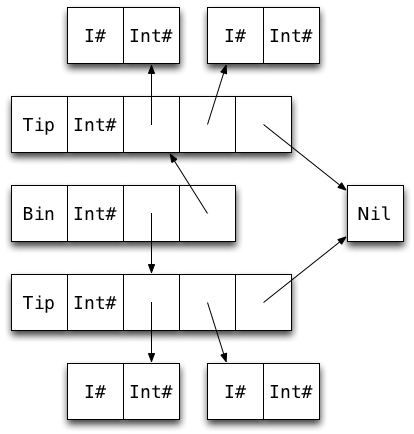22 words. 14 (64%) of them overhead.

In general: 5N + 4(N − 1) words + size of keys & values

# Remaining sources of inefficiency

• Keys and values are still boxed.

• There are quite a few interior nodes. A wider fanning tree would be better. (See the video and slides from my talk at HIW2011.)

A function application is only evaluated if its result is needed, therefore:

• One of the function's right-hand sides will be evaluated.

• Any expression whose value is required to decide which RHS to evaluate, must be evaluated.

These two properties allow us to use "back-to-front" analysis (known as demand/strictness analysis) to figure which arguments a function is strict in.

``max :: Int -> Int -> Intmax x y    | x > y     = x    | x < y     = y    | otherwise = x  -- arbitrary``
• To pick one of the three RHSs, we must evaluate `x > y`.

• Therefore we must evaluate both `x` and `y`.

• Therefore `max` is strict in both `x` and `y`.

# Poll

``data Tree = Leaf | Node Int Tree Treeinsert :: Int -> Tree -> Treeinsert x Leaf   = Node x Leaf Leafinsert x (Node y l r)    | x < y     = Node y (insert x l) r    | x > y     = Node y l (insert x r)    | otherwise = Node x l r``

Which argument(s) is `insert` strict in?

• None

• 1st

• 2nd

• Both

# Solution

Only the second, as inserting into an empty tree can be done without comparing the value being inserted. For example, this expression

``insert (1 `div` 0) Leaf``

does not raise a division-by-zero expression but

``insert (1 `div` 0) (Node 2 Leaf Leaf)``

does.

# Strictness annotations in the real world

``delete :: (Eq k, Hashable k) => k -> HashMap k v -> HashMap k vdelete k0 = go h0 k0  where    h0 = hash k0    go !h !k t@(Bin sm l r)        | nomatch h sm = t        | zero h sm    = bin sm (go h k l) r        | otherwise    = bin sm l (go h k r)    go h k t@(Tip h' l)        | h == h'      = case FL.delete k l of            Nothing -> Nil            Just l' -> Tip h' l'        | otherwise    = t    go _ _ Nil         = Nil{-# INLINABLE delete #-}``
• Without the bang patterns, `go` is not strict in the key `k` or the hash `h` (why?).

• Making `go` strict in the key and hash arguments allows GHC to unbox these values in the loop (after `delete` has been inlined and the key type is known).

# Benchmark

So, is `HashMap` faster than `Map`? Benchmark: 212 random `ByteString` keys of length 8

``````benchmarking Map/lookup/ByteString
mean: 1.590200 ms
std dev: 30.69466 us

benchmarking HashMap/lookup/ByteString
mean: 575.9371 us
std dev: 8.790398 us

benchmarking Map/insert/ByteString
mean: 2.957678 ms
std dev: 451.8105 us

benchmarking HashMap/insert/ByteString
mean: 1.506817 ms
std dev: 301.2400 us
``````

Yes!

# Memory usage

Benchmark: 220 key-value pairs of type `Int`, on a 64-bit machine

Estimated: 8 * (5N + 4(N − 1) + 4N) = 104 MB

Real:

``````   716,158,856 bytes allocated in the heap
1,218,205,432 bytes copied during GC
106,570,936 bytes maximum residency (16 sample(s))
3,636,304 bytes maximum slop
269 MB total memory in use (0 MB lost due to fragmentation)
``````

Maximum residency is the number we care about (note that the value is sampled and thus not 100% accurate).

# Summary

• Focus on memory layout and good performance almost always follows.

• Strictness annotations are mainly used on loop variables and in data type definitions.

• `Data.HashMap` is implemented in the unordered-containers package. You can get the source from http://github.com/tibbe/unordered-containers

# Bonus: memory footprint of some common data types

Write this down on an index card and keep around for back-of-the-envelope calculations.

Data type Memory footprint
`Data.ByteString` 9 words + N bytes
`Data.Text` 6 words + 2N bytes
`String` 5N words
`Data.Map` 6N words + size of keys & values
`Data.Set` 5N words + size of elements
`Data.IntMap` 3N + 5(N-1) words + size of values
`Data.IntSet` 2N + 5(N-1) words
`Data.HashMap` 5N + 4(N-1) words + size of keys & values
`Data.HashSet` 5N + 4(N-1) words + size of elements

(Some caveats apply.)

# Bonus: Use libraries tuned for performance

Non-exhaustive list:

• bytestring - binary data

• text - Unicode data

• containers - data structures

• unordered-containers - more data structures

• vector - mutable/immutable boxed/unboxed vectors

• aeson - JSON serialization/deserialization

• binary - serialization/deserialization of binary data

• attoparsec - parsing of protocols that mix binary and ASCII (e.g. HTTP)